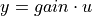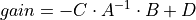# control.matlab.dcgain¶

control.matlab.dcgain(*args)[source]

Compute the gain of the system in steady state

The function takes either 1, 2, 3, or 4 parameters:

Parameters
• A (array-like) – A linear system in state space form.

• B (array-like) – A linear system in state space form.

• C (array-like) – A linear system in state space form.

• D (array-like) – A linear system in state space form.

• Z (array-like, array-like, number) – A linear system in zero, pole, gain form.

• P (array-like, array-like, number) – A linear system in zero, pole, gain form.

• k (array-like, array-like, number) – A linear system in zero, pole, gain form.

• num (array-like) – A linear system in transfer function form.

• den (array-like) – A linear system in transfer function form.

• sys (LTI (StateSpace or TransferFunction)) – A linear system object.

Returns

gain – The gain of each output versus each input:Return type

ndarray

Notes

This function is only useful for systems with invertible system matrix A.

All systems are first converted to state space form. The function then computes: